# Slice String in JavaScript

#### Slice

Slice is nothing but Segment or Division or Part, slice is consider as the one of the method in split string, the slice metod extracts the parts of string and returns the extracted parts in a new string. It has two parameters 'Start' & 'End', in this 'Start' is the required paramater which represents the extraction satrting position and 'End' is the optional parameter which represents the end position of the extraction. In slice method first character has the position '0', second character has position '1' like and so on.....

NOTE: Positive parameters extracts from 'Start' to 'End' and negative parameters extract from 'End' to 'Start'.

`String.slice(start, end)` are the parameters of Slice method.

In JavaScript, by using `slice()` method we can extracts parts of a string and returns the extracted parts in a new string.

EXAMPLE: Extracting the start and end characters in a string.

```<p>Click the below button to extract.</p>
<input type="button" value="Extract" onclick="Function()" />
<p id="myId"></p>

<script>
function Function() {
var a = "TechFunda";
var r = a.slice(1,8);
document.getElementById("myId").innerHTML = r;
}
</script>
```

In the above code snippet we have given `Id` as "myId" to the second `<p>` element, there is a string with the variable `a` in the `<script>` code. In that string value ("TechFunda") we need to extract the "1st" & "8th" parameter, for that we are using string method `.slice(1, 8)`. Onlcick of the button "Extract" in HTML code fires the Function() in the `<script>` code at the same time the string method `slice()` strated extracting the "1st" & "8th" parameter in the string value and returns the extracted parts in new string.

OUTPUT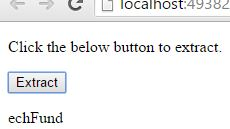EXAMPLE: Extarcting the whole string and returning the full length of the string.

```<p>Click the below button to extract the whole string.</p>
<input type="button" value="Extract" onclick="Function()" />
<p id="myId"></p>

<script>
function Function() {
var a = "TechFunda";
var r = a.slice(0);
document.getElementById("myId").innerHTML = r;
}
</script>
```

Extracting whole string

In the above code snippet we have given value "0" to the `slice()` method for extracting the whole string. Onclick of the button "Extract" in the HTML code fires the Function() in the `<script>` block, at the same time `slice(0)` extracts the whole string and returns the full length of the string as a new string.

OUTPUT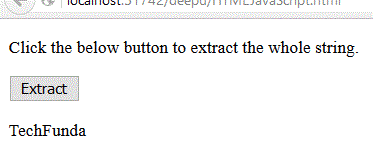EXAMPLE: Extracting the 1st character.

```<p>Click the below button to extract the first character.</p>
<input type="button" value="Extract" onclick="Function()" />
<p id="myId"></p>

<script>
function Function() {
var a = "TechFunda";
var r = a.slice(0, 1);
document.getElementById("myId").innerHTML = r;
}
</script>
```

Extract first character

We need to extract the first character from a string, for that we are using `slice(0, 1)`. Onclick of the button "Extract" in the HTML code fires the Function() in the `<script>` block at the same time `slice(0, 1)` extracts the first character from a string and returns the extracted character as a new string.

OUTPUT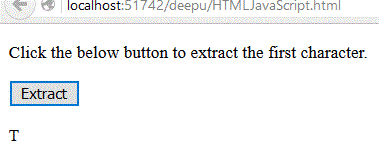EXAMPLE: Extracting the last character.

```<p>Click the below button to extract the last character.</p>
<input type="button" value="Extract" onclick="Function()" />
<p id="myId"></p>

<script>
function Function() {
var a = "TechFunda";
var r = a.slice(-1);
document.getElementById("myId").innerHTML = r;
}
</script>
```

Extract last character

We need to extract the last character of string, for that we are using `slice(-1)`. Onclick of the button extracts the last character from a string and returns the extracted character in a new string.

OUTPUT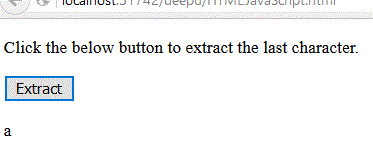EXAMPLE: Extracting from the position '2'.

```<p>Click the below button to extract from the postion 2.</p>
<input type="button" value="Extract" onclick="Function()" />
<p id="myId"></p>

<script>
function Function() {
var a = "TechFunda";
var r = a.slice(2);
document.getElementById("myId").innerHTML = r;
}
</script>
```

Extract from position 2

We need to extract the string from position 2, for that we are using `slice(2)`. Onclick of the button extracts the string from position '2' and returns the extracted part in a new string.

OUTPUT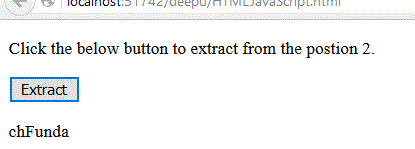EXAMPLE: Extracting starts at '2' and ends at '7'.

```<p>Click the below button to extract from 2 to 7.</p>
<input type="button" value="Extract" onclick="Function()" />
<p id="myId"></p>

<script>
function Function() {
var a = "TechFunda";
var r = a.slice(2, 7);
document.getElementById("myId").innerHTML = r;
}
</script>
```

Extract from 2 to 7

We need to extract the string from position 2 to 7, for that we are using `slice(2, 7)`. Onclick of the button extracts and returns the extracted part in a new string.

OUTPUTViews: 3965 | Post Order: 136